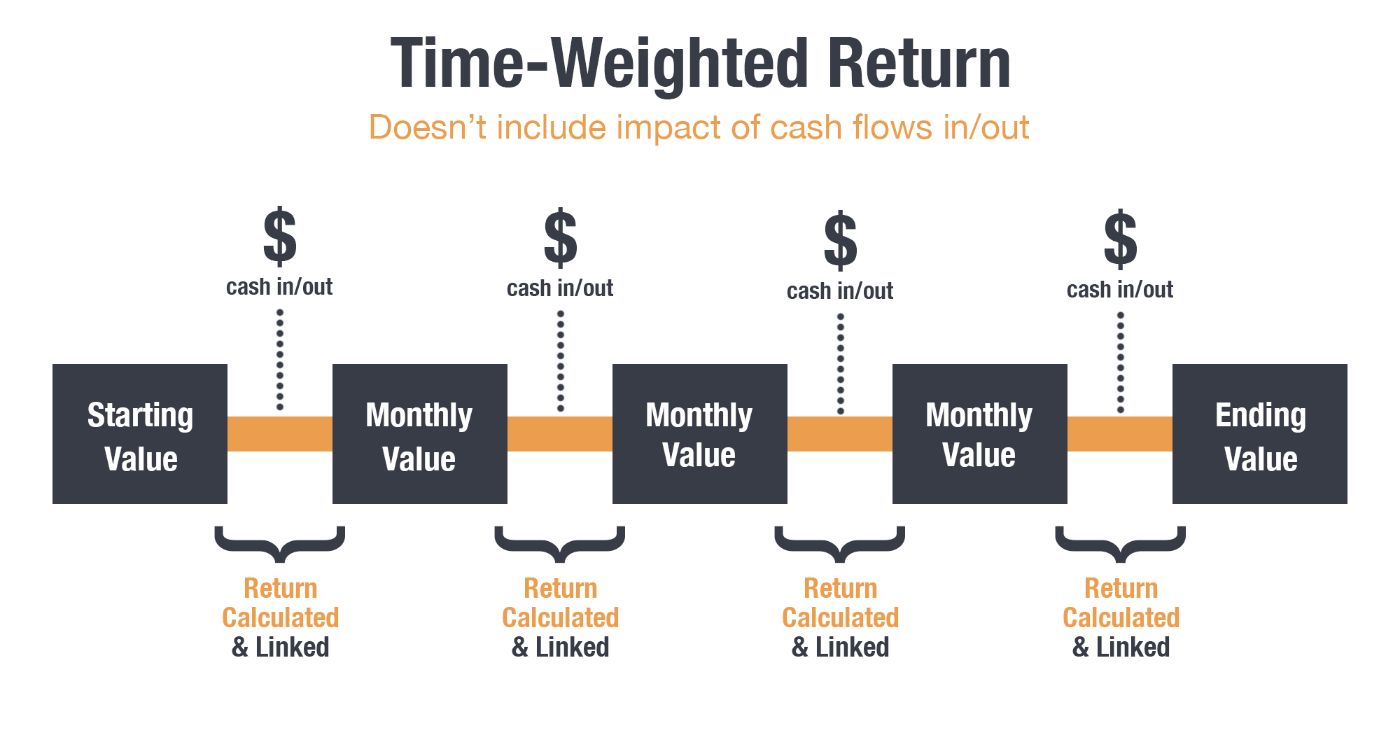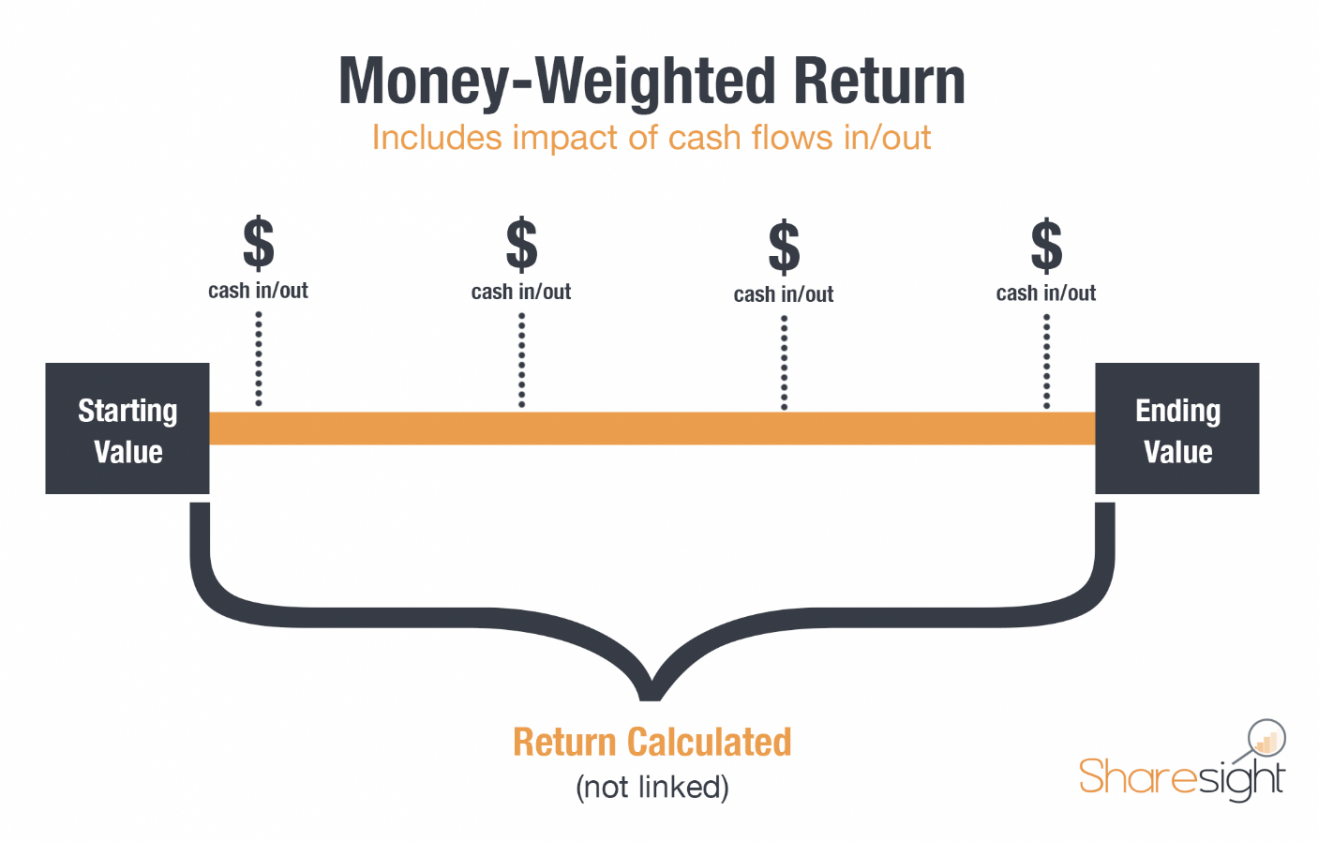# The weighted rate of return: TWRR vs. MWRR

2022.7.8

In this article we will look at the concepts of TWRR and MWRR, i.e. time-weighted rate of return (TWRR) and money-weighted rate of return (MWRR). We are dealing with two measures of return that take into account the capitalization effect. However, there is a big difference between them.

Calculations based on arithmetic return and geometric return, which we analyzed in the article arithmetic return and geometric return, do not consider cash inflows and outflows in the portfolio. And this fact, depending on how it is considered, can result in very different values.

To better understand the problem at hand, let's imagine the following example: an investor invests €10,000 over 3 years. In the first year he invests €8,000 and in subsequent years €1,000 each year. If the first year return is -50% the impact on the portfolio will be huge. Now imagine that in the first year you had invested only €1,000, the effect of this negative return would be significantly smaller.

It can be difficult to determine how much money has been earned in an investment portfolio because deposits and withdrawals influence the return value of the portfolio. Therefore, these metrics should be used to obtain a better assessment of the portfolio.

### First, definitions:

1. Time-weighted return (TWRR): is the compound growth rate of an investment over a given period of time and which does not take into account the impact of cash flows in and out of the portfolio. That is, it is a performance measure of an investment that is not sensitive to the timing and amount of portfolio withdrawals and reinforcements;
2. Money-weighted return (MWRR): It is the growth rate of an investment taking into account all cash inflows and outflows.

### Time Weighted Return (TWRR)

The time-weighted rate of return seeks to neutralize the impact of cash inflows and outflows in a portfolio. It is calculated using the periodic return on investment formula, which we have seen in this article, dividing the investment period into several sub-periods that take into account the value of the portfolio at the moment immediately before the reinforcement or withdrawal. Then, the returns of the different sub-periods are linked to calculate the geometric mean return of the total period. Here, you can see the full definition provided by Investopedia.

It is the preferred performance measure of asset managers and various financial instruments available to invest such as investment funds. The manager generally has no control over the timing and amount of cash flows that enter and leave the portfolio.

To try to simplify and exemplify this concept, follow us in the following scheme, inspired by Sharesight:With the entry or exit of money in the portfolio, the period is divided into smaller periods to calculate the performance of that subperiod which are then combined to calculate the total performance.

Thus, before the entry or exit of money, the value of the portfolio and the respective return are calculated. And so on in the following sub-periods.

### Money weighted return (MWRR)

The cash-weighted return represents the money invested and informs the investor of the return actually earned on their investment. The calculation of the money-weighted return is similar to the internal rate of return and the yield to maturity. As with the calculation of the internal rate of return, invested amounts are treated as outputs from the investor's perspective and amounts returned or withdrawn by the investor, or money remaining at the end of an investment cycle, is an input to the investor. Here you can see the definition of MWRR on investopedia.

Cash outflows:

• The cost of entry into an investment;
• Reinvestment of interest and dividends;
• Surveys.

Cash inflows:

• The values resulting from the sale of an investment;
• Portfolio contributions/reinforcements.Thus, unlike the time-weighted return, which removes the impact of cash flows in the portfolio, this measure calculates the performance of an investment by taking into account the amount and timing of cash inflows and outflows in an investment portfolio.

One of the disadvantages of this method is that it gives greater weight to periods when the portfolio is higher. If an investor reinforces the portfolio before a period of high performance, this reinforcement becomes positive for the portfolio. However, if you raise money before a period of better performance, the impact on performance will be negative.

On the other hand, at the investor level, this is the most appropriate measure, as the investor is usually able to control the moments and amounts of monetary flows.

### Example of application of the two methods – TWR and MWR side by side

The example displays the following facts:

• On January 1, 2022 the portfolio had a value of €100,000
• On April 30, 2022 the portfolio had increased to €150,000
• On 1 May 2022 the portfolio was reinforced by €200,000
• As at 31 December 2022, the portfolio was worth €250,000.

In this scenario, we know that the investor was at a loss of €50,000. What about the TWR and MWR return measures?

TWR (CAGR): 7.1% per year

MWR: -21.0% per year

As can be seen, the results are quite different and the reason is due to the weight of the money invested on May 1, at a time that preceded a period of negative performance.

It is also important to note that the TWR gives a positive value, despite the fact that the investor reached the end of the period with an effective devaluation in the portfolio. This is because this metric neutralizes the effects of cash inflows and outflows. This approach benefited from periodic return on investment prior to reinforcement.

As we could see, there are reasons, benefits and disadvantages in using each of the metrics. The individual investor, with control over the cash inflows and outflows of his portfolio, must use the MWR as a performance measure. However, as the TWR is the metric most used by asset managers and management companies, it is also important and valid for the purpose of comparing the various actors and financial instruments under analysis.Vítor Ribeiro, CFA

Vítor is a CFA® charterholder, entrepreneur, music lover and with a dream of building a true investment and financial planning ecosystem at the service of families and organizations.

+351 939873441 (Vítor Mário Ribeiro, CFA)

+351 938438594 (Luís Silva)

future@futureproof.pt

Future Proof is an Appointed Representative of Banco Invest, S.A.. It is registered at CMVM.